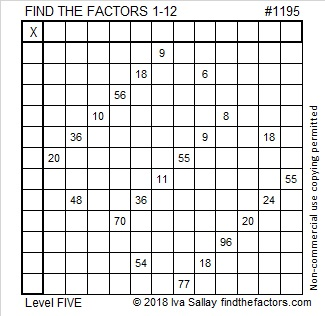# 1195 You Can Find the Answer in This Book

The new school year is underway. Much may have been forgotten over the summer. If you don’t quite remember all the multiplication tables, this puzzle book can help you remember them AND help your brain grow. You might still find it a challenge, but that only makes it more fun!Print the puzzles or type the solution in this excel file: 12 factors 1187-1198

Now I’ll share a few facts about the number 1195:

• 1195 is a composite number.
• Prime factorization: 1195 = 5 × 239
• The exponents in the prime factorization are 1 and 1. Adding one to each and multiplying we get (1 + 1)(1 + 1) = 2 × 2 = 4. Therefore 1195 has exactly 4 factors.
• Factors of 1195: 1, 5, 239, 1195
• Factor pairs: 1195 = 1 × 1195 or 5 × 239
• 1195 has no square factors that allow its square root to be simplified. √1195 ≈ 34.568771195 is also the hypotenuse of a Pythagorean triple:
717-956-1195 which is (3-4-5) times 239